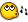## Recommended Posts

Ok I can't make sense of the way color is written in autoit. I thought decimal is written seperately for red, green and blue.

I'm trying to determine the color difference between what my screencapture software sees and what I actually have in my game. Here are some examples:

Screencap sees: 13103350

Game Script sees: 11593960

another example,

Screencap sees: 4325619

Game Script sees: 2162911

If I can determine the difference maybe I can find the true color without having to run my getcolor script to tell me what the real color is. I can't make head or tails of this color writing format so any help is greatly appreciated.

##### Share on other sites

Are you using the same color depth (such as 16-bit and 32-bit) in both cases? Also make sure images are in a format like bmp (instead of jpeg which can have color distorations).

To convert from decimal to rgb:

Convert the decimal number to a 6-digit hexadecimal number.

Mark off the number in groups of two digits.

The colors are blue, green, and red.

Convert the hex back to decimal.

Example: 13103350

in hex is, C7 F0 F6

so red = F6, green = F0, and blue = C7

or RGB = (246, 240, 199)

Likewise: 11593960

hex is, B0 E8 E8

r = E8, g = E8, b = B0

RGB = (232, 232, 176)

If my calcualtions are corret, the RGB values show that the colors are quite similar. Make sure you are getting the correct pixel instead of one next to it.

Hope that helps

Edited by CyberSlug

Use Mozilla | Take a look at My Disorganized AutoIt stuff | Very very old: AutoBuilder 11 Jan 2005 prototype I need to update my sig!

##### Share on other sites

Also a function / example:\$pix = MouseGetPos()

\$var = PixelGetColor( \$pix, \$pix)

\$hex= hex(\$var, 6)

;// Arranging the AutoIt output ¿?

\$hexarr= stringright(\$hex, 2) & stringmid(\$hex, 3, 2) & stringleft(\$hex, 2)

msgbox(0, "Example", "HEX: #" & \$hexarr & @LF & "RGB= " & RGB(\$hex))

exit

; Returns the RGB (Red, Green, Blue) values

Func RGB(\$hex)

Local \$val

Local \$rgb

\$val= StringLower(\$hex)

Dim \$v

For \$i=0 to 6

\$v[\$i]= GDec(stringmid(\$val, \$i+1, 1))

Next

\$x= (\$v * 16) + \$v

\$y= (\$v * 16) + \$v

\$z= (\$v * 16) + \$v

;; arrange

\$rgb= "(" & \$z & ", " & \$y & ", " & \$x & ")"

Return \$rgb

EndFunc

;// Convert HEX to DEC

Func GDec(\$hex)

Local \$n

Local \$hex

\$n= \$hex

Select

Case \$hex == "a"

\$n= 10

Case \$hex == "b"

\$n= 11

Case \$hex == "c"

\$n= 12

Case \$hex == "d"

\$n= 13

Case \$hex == "e"

\$n= 14

Case \$hex == "f"

\$n= 15

EndSelect

Return \$n

EndFunc

##### Share on other sites

The last few versions support colours in hex as well. AutoIt Spy gives the colours in dec and hex and for the Pixel functions where it says "decimal colour" you can use hex (although I thought I'd changed the docs, maybe I only changed the docs in the current .92 unstable version)

The PixelGetColor function returns a number, you can convert this to a hex string using either the Hex() function or StringFormat(). Or you can even just use BitAnd() to mask off the bits you are interested in.

That reminds me, I need to add the bit shifts that tylo suggested.

## Create an account

Register a new account

• ### Recently Browsing   0 members

×

• Wiki

• Back

• #### Beta

• Git
• FAQ
• Our Picks
×
• Create New...